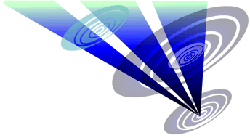Reconstruction of the 2019 Cocum Farm formation 1.Draw a circle. Draw the horizontal and vertical centerlines. 2.Construct the inscribed equilateral triangle of circle 1, pointing up. 3.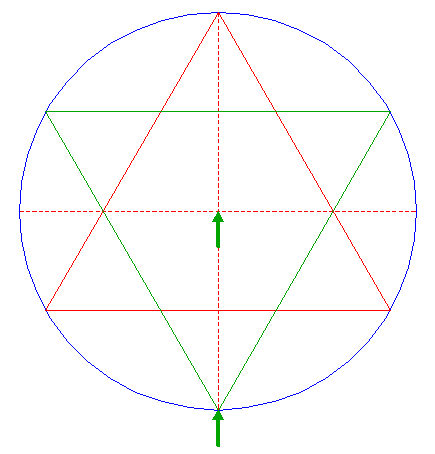Construct the inscribed equilateral triangle of circle 1, pointing down. 4.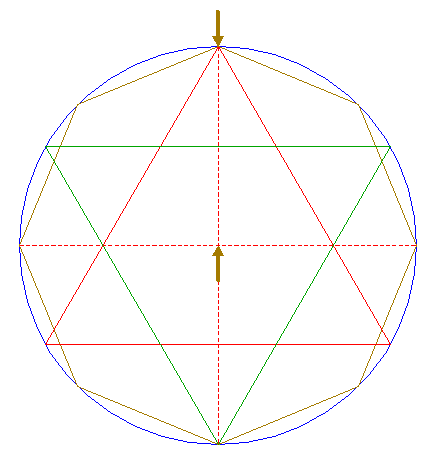Construct the inscribed octagon (regular 8-sided polygon) of circle 1, pointing up. 5.Construct the inscribed circle of octagon 4. 6.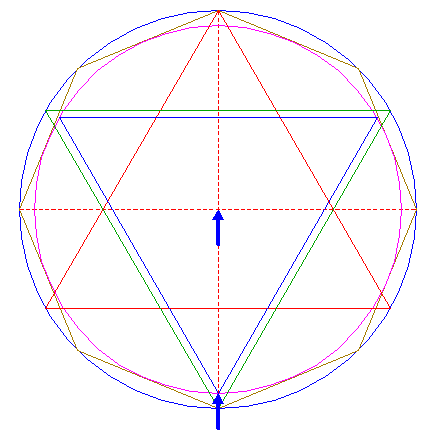Construct the inscribed equilateral triangle of circle 5, pointing down. 7.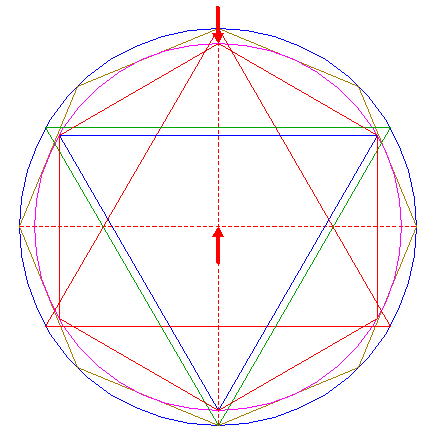Construct the inscribed hexagon (regular 6-sided polygon) of circle 5, pointing up. 8.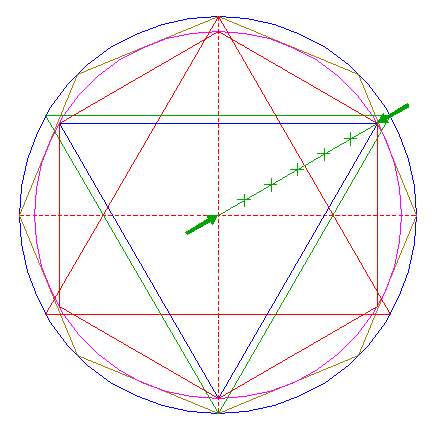Draw the ray of circle 1 to the upper righthand angular point of triangle 6. Divide this line into six equal parts. 9.Construct five circles centered at the upper righthand angular point of triangle 6, passing through dividing nodes 8. 10.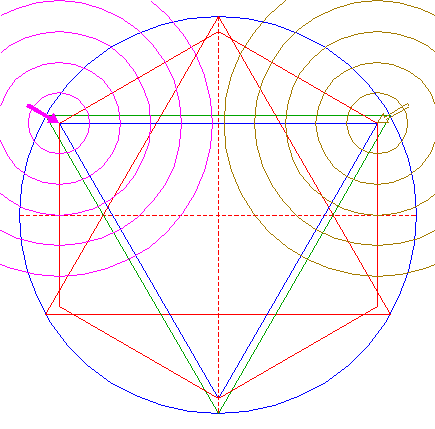Copy circles 9 to the upper lefthand angular point of triangle 6. 11.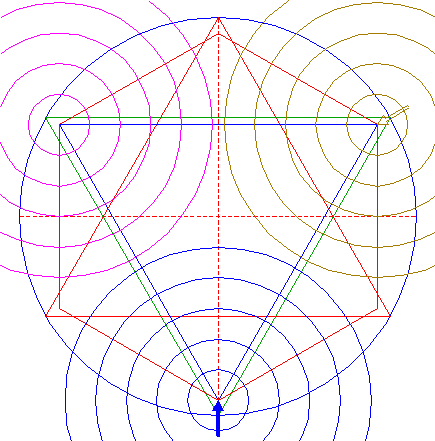Copy circles 9 to the lower angular point of triangle 6. 12.Draw four connecting lines, parallel to the upper side of triangle 6, between the intersections of circles 9 and the righthand side, and the intersections of circles 10 and the lefthand side of triangle 6, except for the outer circles. 13.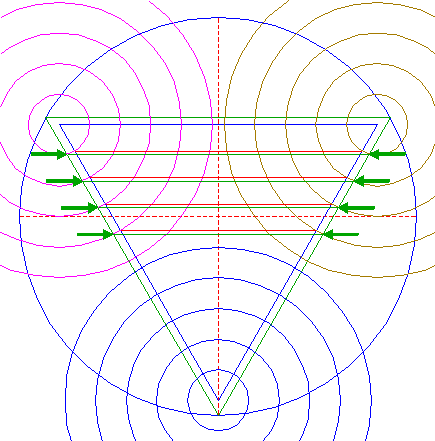Draw four connecting lines, parallel to the upper side of triangle 3, between the intersections of circles 9 and the righthand side, and the intersections of circles 10 and the lefthand side of triangle 3, except for the outer circles. 14.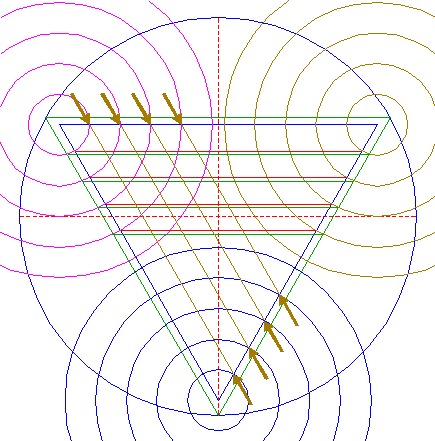Draw four connecting lines, parallel to the lefthand side of triangle 6, between the intersections of circles 10 and the upper side, and the intersections of circles 11 and the righthand side of triangle 6, except for the outer circles. 15.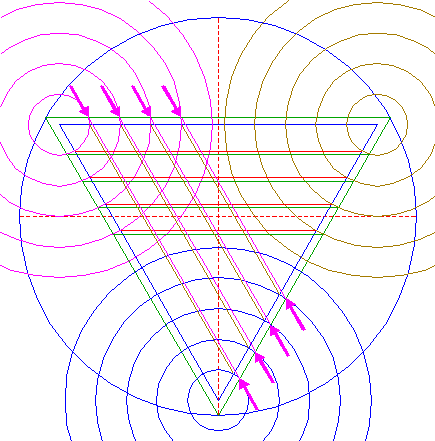Draw four connecting lines, parallel to the lefthand side of triangle 3, between the intersections of circles 10 and the upper side, and the intersections of circles 11 and the righthand side of triangle 3, except for the outer circles. 16.Draw four connecting lines, parallel to the righthand side of triangle 6, between the intersections of circles 9 and the upper side, and of circles 11 and the lefthand side of triangle 6, except for the outer circles. 17.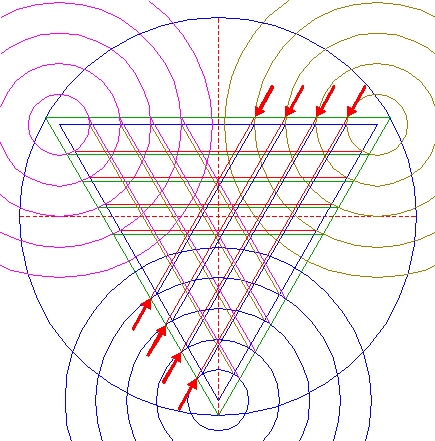Draw four connecting lines, parallel to the righthand side of triangle 3, between the intersections of circles 9 and the upper side, and the intersections of circles 11 and the lefthand side of triangle 3, except for the outer circles. 18.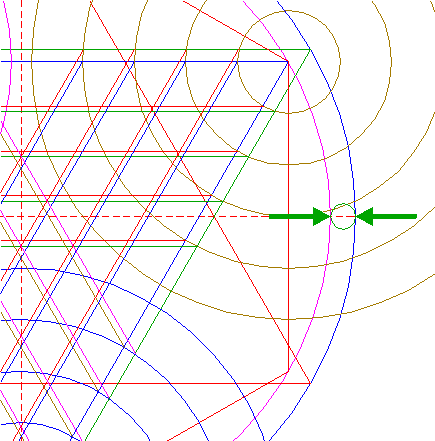Construct a "two-points" circle (defined by the two end-points of a centerline) between the righthand intersections of circles 1 and 5 with the horizontal centerline. 19.Construct a circle concentric to circle 1, passing through the center of circle 18. 20.Circles 9, 10, 11, and 19, triangles 2, 3, and 6, hexagon 7, and lines 12, 13, 14, 15, 16, and 17, are used for the final reconstruction. 21.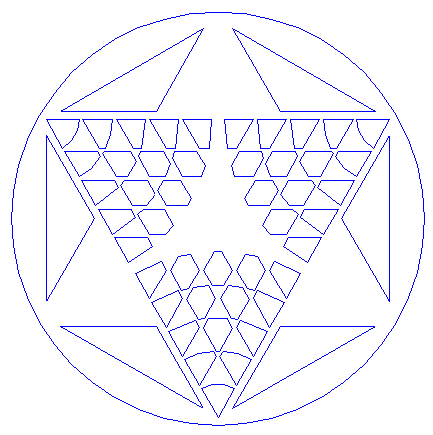Remove all parts not visible within the formation itself. 22.Colour all areas corresponding to standing... 23....or to flattened crop, and finish the reconstruction of the 2019 Cocum Farm formation. 24.The final result, matched with the aerial image.